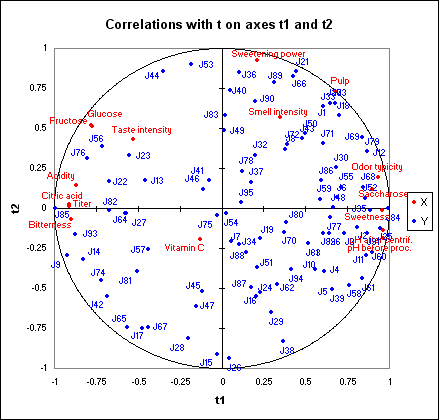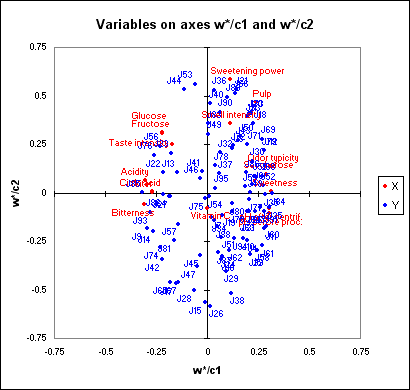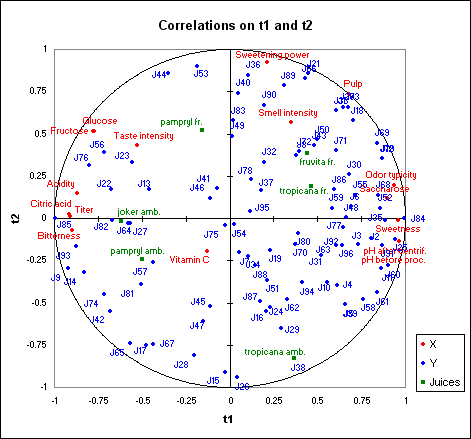# Partial Least Squares PLS regression in Excel

2018-12-03

This tutorial will help you set up and interpret a Partial Least Squares regression in Excel using the XLSTAT software.
Not sure this is the modeling feature you are looking for? Check out this guide

## Dataset for running a Partial Least Squares regression

This tutorial is based on data that have been extensively analyzed in [Tenenhaus, M., Pagès, J., Ambroisine L. and & Guinot, C. (2005); PLS methodology for studying relationships between hedonic judgments and product characteristics; Food Quality an Preference. 16, 4, pp 315-325].

The data used in this article correspond to 6 orange juices described by 16 physico-chemical descriptors and evaluated by 96 judges.

An Excel sheet with both the data and the results can be downloaded by clicking on the button below:

## Goal of the Partial Least Squares regression in this example

Partial Least Squares regression is going to allow us to obtain a simultaneous map of the judges, the descriptors, and the products, and then to analyze for some judges which descriptors are related to their preferences.

## Setting up a Partial Least Squares regression

To activate the Partial Least Squares regression dialog box, start first XLSTAT, then select the XLSTAT / Modeling data / Partial Least Squares Regression function.Once you have clicked the button, the Partial Least Squares regression dialog box is displayed.

In the Dependent variable(s) field, select with the mouse the ratings of the 96 judges.

The ratings are the "Ys" of the model as we want to explain the ratings given by the judges.

In the Quantitative variable(s) field, select the explanatory variables, that are in our case the physicochemical descriptors.

The name of the orange juices has also been selected as Observation labels.

In the Options tab of the dialog box, we fix the number of components at 4 in the Stop conditions.

Last, in the Charts tab, the Colored labels option has been activated in order to make the reading of the charts easier. The Vectors option has been unchecked in order not to saturate the charts.The extremely fast computations start when you click on OK. The display of the results is stopped to allow you to select the axes for the maps.You only need to click on "Done" so that the charts are only displayed for the first two axes.

## Interpreting the results of a Partial Least Squares regression

The display of the results may take few seconds as there are many tables and charts because of the 96 dependent variables.

After the tables displaying the basic statistics and the correlations between all the selected variables (dependent variables are displayed in blue and quantitative explanatory variables in black), the results specific to the PLS regression are presented.

The first table and the corresponding bar chart allow visualizing the quality of the Partial Least Squares regression as a function of the number of components.The Q² cumulated index measures the global goodness of fit and the predictive quality of the 96 models.

We see that Q² remains low even with 4 components (ideally it should be close to 1). This suggests that the quality of the fit varies a lot depending on the judge.

The cumulated R²Y and R²X cum that corresponds to the correlations between the explanatory (X) and dependent (Y) variables with the components are very close to 1 with 4 components. This indicates that the 4 components generated by the Partial Least Squares regression summarize well both the Xs and the Ys.

The first correlations map allows visualizing on the first two components the correlations between the Xs and the components, and the Ys and the components.We can see that for some judges displayed at the center of the map, the correlations are low. By looking at the corresponding table, we see that for example, the J54 judge is only correlated with the fourth component, that is globally little correlated with the explanatory variables.

Regarding the explanatory variables, we notice that the Vitamin C is not well represented on the first two dimensions. We can interpret this as the fact that this variable explains only little the preferences of the judges, which is not surprising as it does not have a strong effect on taste or other criteria that could easily influence the judges' preference. We notice the strong correlations between the fructose and the glucose, between the two pH, and the negative correlation between the pH and the acidity and titer. One should also notice how different the judges are: they are not concentrated on one part of the correlations circle, but well distributed all around it.

The map that displays the dependent variables on the c vectors and the explanatory variables on the w* vectors allows visualizing the global relationship between the variables. The w* are related to the weights of the variables in the models.If one projects an explanatory variable on the vector of a dependent variable (the vectors are displayed only if there are less than 50 dependent variables) we have an idea of the influence of the explanatory variable in the modeling of the dependent variable.

The coordinates of the orange juices in the space of the t coordinates are available on a table and displayed on a map. We notice that the products are well distinguished.A new correlations map allows superimposing the products on the previous correlations map. In the legend, we replaced "Obs" by "Juices", by modifying the series name in the Excel toolbar, after we selected the series by clicking on one of the points. As almost always with XLSTAT, the charts are Excel charts and can easily be modified.In their article, Tenenhaus et al. interpret this chart in detail. They deduce from it the existence of 4 well-identified clusters of judges. They advise re-running Partial Least Squares regression on each of these groups. This way they obtain better Q² and R². For the first group, the R²Y is 0.63 instead of the 0.53 we obtained with all the judges.

Two tables giving results for the u and u~ components are then displayed. A chart allows visualizing the observations (in our case the juices) in the space of the u~.

The following tables allow visualizing for each dependent variable the Q² et cumulated Q² indexes as a function of the number of components. We notice that for several variables, the maximum of the cumulated Q² is obtained for one or two components only (for example J5, J6, J7).

A series of tables with the R² for each entry variable with the t components is optionally displayed. The option is not activated by default, and the tables are not taken into account in this tutorial.

The following table displays the VIPs (Variable Importance for the Projection) for each explanatory variable, for an increasing number of components. This allows to quickly identify which are the explanatory variables that contribute the most to the models. For the model with one component, we can see that the Vitamin C, the Sweetening power, the Odor intensity and the Taste intensity have a low influence on the models.The next table displays the parameters (or coefficients) of the models corresponding to each dependent variable. The equations of the models are displayed below that table. The equations can be later reused for simulation or prediction purposes.

For each model, XLSTAT displays the goodness of fit coefficients, the standardized coefficients table, and the table of predictions and residuals. The analysis of the model corresponding to judge J1 allows concluding that the model is well fitted (R’² equals 0.88). However, the number of degrees of freedom is low and might be facing an overfitting problem. This is also confirmed when we look at the standardized coefficients: for each coefficient, the confidence intervals are wide and include 0. As we noticed that the cumulated Q’² corresponding to this model reaches its maximum value with 2 components, it is likely that a model with only two components would be better.

We have performed a new Partial Least Squares regression analysis, using just J1 as a dependent variable, and forcing the number of components (see the options tab) to 2. The results are displayed on the PLS2 sheet. We can now see that the quality of the results has been improved. The following chart corresponds to the standardized coefficients of the new model.We can see here that the coefficients are significantly different from zero only for "Smell intensity" and "Odor typicity". The predictions and residuals table allows verifying that the rates given by the judge 1 are well reproduced by the model.

Last, the table with the DModX and DModY, and the corresponding charts that allow to quickly identify potential outliers, are displayed. In our case, there aren't any outlier as all values are lower than DCritX or DCritY.

#### Contact our technical support team: support@xlstat.com

https://cdn.desk.com/
false
desk